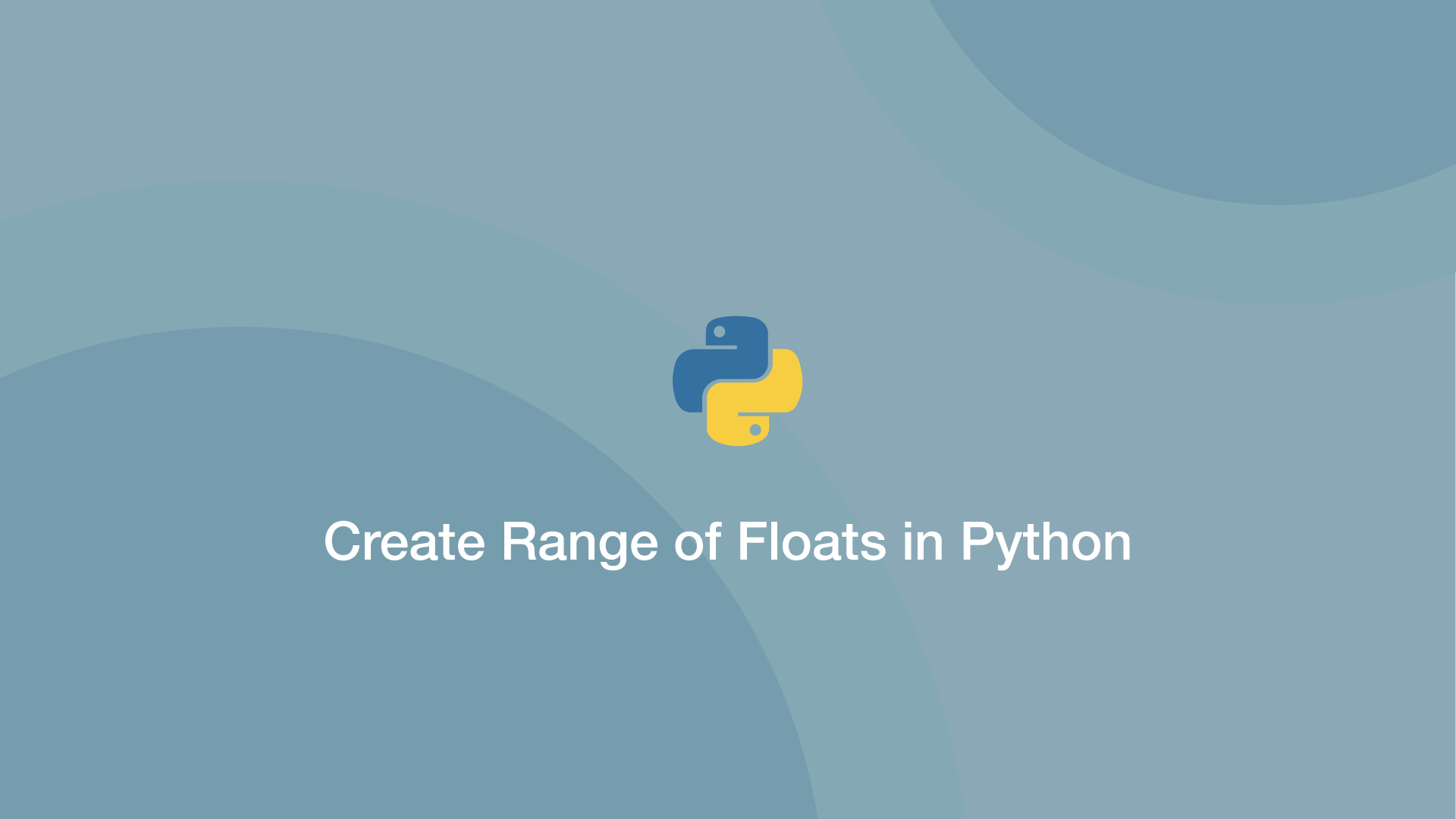# How to Create Range of Floats in PythonYou'll notice when attempting to create a range of floats in Python like this:

``````for i in range(0, 1, 0.1):
print(i)
``````

The following `TypeError` is returned:

``````TypeError: 'float' object cannot be interpreted as an integer
``````

In this tutorial, we will show some of the different ways to create ranges of floats in Python that work.

## NumPy Arange

NumPy contains the `arange()` function, which can create a range of numbers in customisable steps.

You may need to install NumPy, which is done using pip:

``````pip install numpy
``````

### NumPy Arange Syntax

``````np.arange (start, stop, step)
``````

The first argument is the first number in the range, the second is number to finish before and the last is the size of the increment between each number.

Let's say we want to create a list of floats from 0.1 to 0.9, we would use NumPy `arange()` like this:

``````import numpy as np

numbers = np.arange(0.1, 1, 0.1)

print(numbers)
``````
``````[0.1 0.2 0.3 0.4 0.5 0.6 0.7 0.8 0.9]
``````

To increment in steps of 0.2, we would need to change the third argument like this:

``````import numpy as np

numbers = np.arange(0.1, 1, 0.2)

print(numbers)
``````
``````[0.1 0.3 0.5 0.7 0.9]
``````

## Range of Floats with List Comprehension

Using Python list comprehension we can divide integers into floats as they are added to a new list.

Here is an example of building a list of numbers from 0.1 to 0.9:

``````foo = [(x / 10 ) for x in range(0, 10)]

print(foo)
``````
``````[0.0, 0.1, 0.2, 0.3, 0.4, 0.5, 0.6, 0.7, 0.8, 0.9]
``````

## Float Range of Tuples Example

We can use Python list comprehension to create a list of tuples containing floating-point numbers. In the example below, tuples containing numbers from 0.1 to 0.10 are created:

``````foo = [(x / 10 ) for x in range(0, 11)]

print(foo)
``````
``````[0.0, 0.1, 0.2, 0.3, 0.4, 0.5, 0.6, 0.7, 0.8, 0.9, 1.0]
``````

#### Related Tutorials### How to Generate a Range of Floats in Python

April 22, 2021### How to Generate Random Numbers in Python

September 20, 2020### How to Use Argparse in Python

September 25, 2020### How to Calculate Mean, Median, Mode and Range in Python

October 07, 2020### The Python Sleep Function

October 01, 2020### How to Round Numbers Up and Down in Python

September 28, 2020